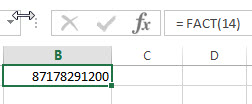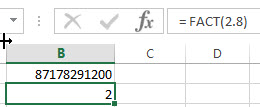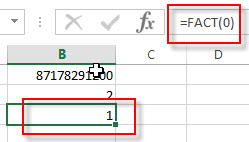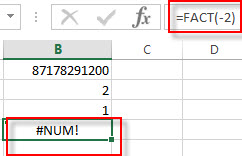# Excel FACT Function

This post will guide you how to use Excel FACT function with syntax and examples in Microsoft excel.

### Description

The Excel FACT function returns the factorial of a given number.  The factorial of a given number is equal to 1*2*3…* number.

The FACT function is a build-in function in Microsoft Excel and it is categorized as a Math and Trigonometry Function.

The FACT function is available in Excel 2016, Excel 2013, Excel 2010, Excel 2007, Excel 2003, Excel XP, Excel 2000, Excel 2011 for Mac.

### Syntax

The syntax of the FACT function is as below:

`= FACT (number)`

Where the FACT function arguments is:

• number – This is a required argument. A numeric value that you want to calculate the factorial. And the number need to be a positive integer. If the number is not an integer and it will be truncated.

Note:

• If the number argument is negative, the FACT function will return #NUM! Error.
• If the number argument is non-numeric, the FACT function will return #VALUE! Error.

### Excel FACT Function Examples

The below examples will show you how to use Excel FACT Function to calculate the natural logarithm of a given number.

1# to get the factorial of number 14, enter the following formula in Cell B1.

`= FACT(14)`2# to get the factorial of the integer of 2.8, enter the following formula in Cell B2.

`= FACT(2.8)`3# to get the factorial of 0, enter the following formula in Cell B3.

`= FACT (0)`4# to get the factorial of a negative number, and returns a #NUM! Error, enter the following formula in Cell B4

`=FACT(-2)`